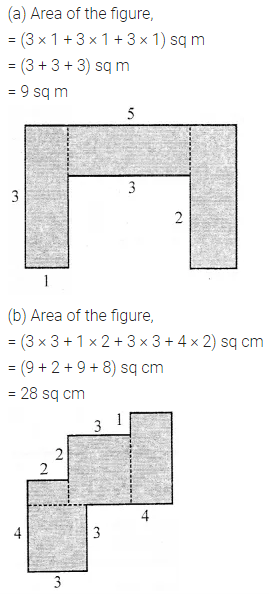ML Aggarwal Class 6 Solutions Chapter 14 Mensuration Check Your Progress for ICSE Understanding Mathematics acts as the best resource during your learning and helps you score well in your exams.

## ML Aggarwal Class 6 Solutions for ICSE Maths Chapter 14 Mensuration Check Your Progress

Question 1.
Look at the given figure and fill in the following blanks: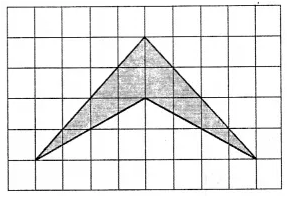(i) The contribution in the estimation of the given area due to completely covered squares is
(ii) The contribution in the estimation of the given area due to more than half covered squares is
(iii) The contribution in the estimation of the given area due to the exactly half-covered square is
(iv) The contribution in the estimation of the given area due to less than half covered squares is
(v) The total estimated area is
Solution:Question 2.
The perimeter of a square ABCD is twice the perimeter of ∆PQR. Find the area of the square ABCD.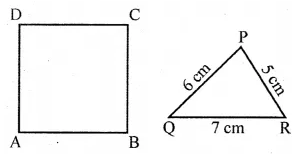Solution:Question 3.
A wire is in the shape of a square of side 10 cm. If the wire is rebent into a rectangle of length 12 cm, find its breadth. Which encloses more area, the square or the rectangle and by how much?
Solution: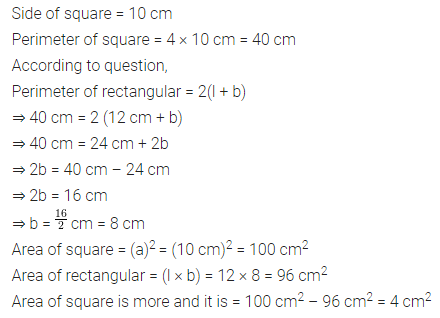Question 4.
A rectangular room is 9 m long and 6 m wide. Find the cost of covering the floor with carpet 2 m wide at ₹35 per metre.
Solution:Question 5.
If the cost of fencing a square plot at the rate of ₹ 2.50 per metre is ₹ 200, then find the length of each side of the field.
Solution: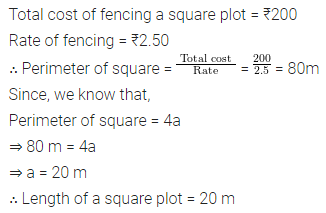Question 6.
If the cost of fencing a rectangular park at the rate of ₹7.50 per metre is ₹600 and the length of the park is 24 m, find the breadth of the park.
Solution: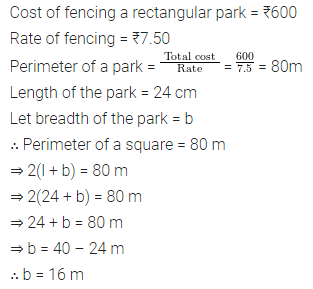Question 7.
By splitting the following figures into a rectangle, find their areas (The measures are given in centimetres).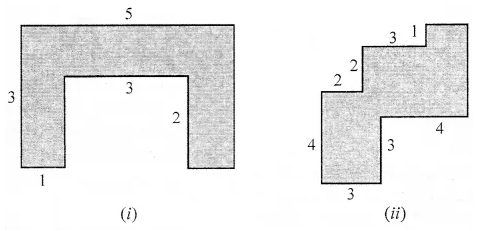Solution: